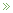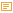• ## Artificial Intuition

Posted by Konstantin 07.12.2008 7 Comments

### Logic versus Statistics

Consider the two algorithms presented below.

Algorithm 1:

   If, for a given brick B,
B.width(cm) * B.height(cm) * B.length(cm) > 1000
Then the brick is heavy

Algorithm 2:

   If, for a given male person P,
P.age(years) + P.weight(kg) * 4 - P.height(cm) * 2 > 100
Then the person might have health problems

Note that the two algorithms are quite similar, at least from the point of view of the machine executing them: in both cases a decision is produced by performing some simple mathematical operations with a given object. The algorithms are also similar in their behaviour: both work well on average, but can make mistakes from time to time, when given an unusual person, or a rare hollow brick. However, there is one crucial difference between them from the point of view of a human: it is much easier to explain how the algorithm "works'' in the first case, than it is in the second one. And this is what in general distinguishes traditional "logical" algorithms from the machine learning-based approaches.

Of course, explanation is a subjective notion: something, which looks like a reasonable explanation to one person, might seem incomprehensible or insufficient to another one. In general, however, any proper explanation is always a logical reduction of a complex statement to a set of "axioms". An "axiom" here means any "obvious" fact that requires no further explanations. Depending on the subjective simplicity of the axioms and the obviousness of the logical steps, the explanation can be judged as being good or bad, easy or difficult, true or false.

Here is, for example, an explanation of Algorithm 1, that would hopefully satisfy most readers:

• The volume of a rectangular object can be computed as its width*height*length. (axiom, i.e. no further explanation needed)
• A brick is a rectangular object. (axiom)
• Thus, the volume of a brick can be computed as its width*height*length. (logical step)
• The mass of a brick is its volume times the density. (axiom)
• We consider the density of a brick to be at least 1g/cm3 and we consider a brick heavy if it weighs at least 1 kg. (axiom)
• Thus a brick is heavy if its mass > width*height*length > 1000. (logical step, end of explanation)

If you try to deduce a similar explanation for Algorithm 2 you will probably stumble into problems: there are no nice and easy "axioms" to start with, unless, at least, you are really deep into modeling body fat and can assign a meaning to the sum of a person's age with his weight. Things become even murkier if you consider a typical linear classification algorithm used in OCR systems for deciding whether a given picture contains the handwritten letter A or not. The algorithm in its most simple form might look as follows:

   If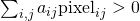Then there is a letter A on the picture,

where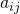are some real numbers that were obtained using an obscure statistical procedure from an obscure dataset of pre-labeled pictures. There is really no good way to explain why the values ofare what they are and how this algorithm gets the result, other than to present the dataset of pictures it was trained upon and state that "well, these are all pictures of the letter A, therefore our algorithm detects the letter A on pictures".

Note that, in a sense, such an "explanation" uses each picture of the letter A from the training set as an axiom. However, these axioms are not the kind of statements we used to justify Algorithm 1. The evidence they provide is way too weak for traditional logical inferences. Indeed, the fact that one known image has a letter A on it does not help much in proving that some other given image has an A too. Yet, as there are many of these "weak axioms", one statistical inference step can combine them into a well-performing algorithm. Notice how different this step is from the traditional logical steps, which typically derive each "strong" fact from a small number of other "strong" facts.

So to summarize again: there are two kinds of algorithms, logical and statistical.
The former ones are derived from a few strong facts and can be logically explained. Very often you can find the exact specifications of such algorithms in the internet. The latter ones are based on a large number of "weak facts" and rely on induction rather than logical (i.e. deductive) explanation. Their exact specification (e.g. the actual values for the parametersused in that OCR classifier) does not make as much general sense as the description of classical algorithms. Instead, you would typically find general principles for constructing such algorithms.

### The Human Aspect

What I find interesting, is that the mentioned dichotomy stems more from human psychology than mathematics. After all, the "small" logical steps as well as the "big" statistical inference steps are all just "steps" from the point of view of maths and computation. The crucial difference is mainly due to a human aspect. The logical algorithms, as well as all of the logical decisions we make in our life, is what we often call "reason" or "intelligence". We make decisions based on reasoning many times a day, and we could easily explain the small logical steps behind each of them. But even more often do we make the kind of reason-free decisions that we call "intuitive". Take, for example, visual perception and body control. We do these things by analogy with our previous experiences and cannot really explain the exact algorithm. Professional intuition is another nice example. Suppose a skilled project manager says "I have doubts about this project because I've seen a lot of similar projects and all of them failed". Can he justify his claim? No, no matter how many examples of "similar projects" he presents, none of them will be considered as reasonable evidence from the logical point of view. Is his decision valid? Most probably yes.

Thus, the aforementioned classes of logical (deductive) and statistical (inductive) algorithms seem to directly correspond to reason and intuition in the human mind. But why do we, as humans, tend to consider intuition to be inexplicable and thus make "less sense" than reason? Note that the formal difference between the two classes of algorithms is that in the former case the number of axioms is small and the logical steps are "easy". We are therefore capable of representing the separate axioms and the small logical steps in our minds somehow. However, when the number of axioms is virtually unlimited and the statistical step for combining them is way more complicated, we seem to have no convenient way of tracking them consciously due to our limited brain capacity. This is somewhat analogous to how we can "really understand" why 1+1=2, but will have difficulties trying to grasp the meaning of 121*121=14641. Instead, the corresponding inductive computations can be "wired in" to the lower, unconscious level of our neural tissue by learning patterns from experience.

### The Consequences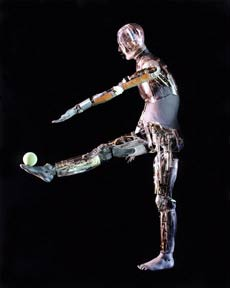There was a time at the dawn of computer science, when much hope was put in the area of Artificial Intelligence. There, people attempted to devise "intelligent" algorithms based on formal logic and proofs. The promise was that in a number of years the methods of formal logic would develop to such heights, that would allow computer algorithms to attain "human" level of intelligence. That is, they would be able to walk like humans, talk like humans and do a lot of other cool things that we humans do. Half a century has passed and this still didn't happen. Computer science has seen enormous progress, but we have not found an algorithm based on formal logic that could imitate intuitive human actions. I believe that we never shall, because devising an algorithm based on formal logic actually means understanding and explaining an action in terms of a fixed number of axioms.

Firstly, it is unreasonable to expect that we can precisely explain much of the real world, because, strictly speaking, there exist mathematical statements that can't in principle be explained. Secondly, and most importantly, this expectation contradicts the assumption that most "truly human" actions are intuitive, i.e. we are simply incapable of understanding them.

Now what follows is a strange conclusion. There is no doubt that sooner or later computers will get really good at performing "truly human" actions, the trend is clear already. But, contradictory to our expectations, the fact that we shall create a machine that acts like a human will not really bring us closer to understanding how "a human" really "works". In other words, we shall never create Artificial Intelligence. What we are creating now, whether we want it or not, is Artificial Intuition.

### Calendar

December 2008
M T W T F S S
« Nov   Jan »
1234567
891011121314
15161718192021
22232425262728
293031